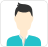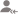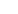true

Find the best tutors and institutes for Class 9 Tuition

Find Best Class 9 Tuition

No matching category found.

No matching Locality found.

Outside India?

Search for topicsPost a Lesson

All

All

Lessons

Discussion

Lesson Posted on 12 JunGursimran kaur

I have an experience of more than 3 years being a tuition teacher of class 9th and 10th.

It is an elementary and exciting chapter in class 9. You can note this lesson as your revision notes as well. You see several things in this universe, what do you think this universe is made up of? It is made up of matter. ALL ABOUT MATTER AND ITS NATURE The matter is anything and everything that... read more

It is an elementary and exciting chapter in class 9. You can note this lesson as your revision notes as well.

You see several things in this universe, what do you think this universe is made up of? It is made up of matter.

ALL ABOUT MATTER AND ITS NATURE

• The matter is anything and everything that occupies space and has mass. It also offers resistance, that is why we need to apply some kind of force while doing any particular task.
• Matter can be felt by our five senses, ignoring/eliminating all the emotions because that neither occupies any space nor has any mass.
• Some examples are mountains, table, milk, the smell of perfume etc.

NOTE: vacuum is not considered to be a matter because there are no particles; hence nothing is occupying space and mass.

Coming on to the characteristics/properties of matter or nature/behaviour of matter:

1) The matter is particulate, i.e., and it is made up of small particles called atoms.

2) The particles of matter are so small that our eyes cannot observe them.

3) The particles of matter are continuously moving.

4) The particles of matter have space between them known as intermolecular space

5) The particles of matter are attracted to each other by the force of attraction, also known as the intermolecular force of attraction.

EXPERIMENTS

All the characteristics and properties can be observed through some easy and straightforward experiments:

Experiment 1: You must have observed that whenever salt is dissolved in water, the level of water does not rise. Have you ever wondered why? Because the particles of water have space between them, which when salt is added, is taken by the particles of salt resulting in no rise in the level of water.

By this experiment, you can conclude that particles of matter have space between them and also the particles of matter are constantly/continuously moving.

Experiment 2: Now comes the concept of DIFFUSION. It is the process of intermixing of particles into each other. Giving some examples through small experiments and observations, Take crystals of potassium permanganate (purple). When you try to mix or dissolve it in water the force if the attraction of the particles of crystals weakens which leads to the particle diffusion and then the particles of crystals dissolves in the water after which the water solution looks purple.

Another example can be an incense stick(Agarbatti). The smell of lit incense stick diffuses into the whole room, why? Because the particles of incense stick intermix with the particles present in the air. Also, diffusion becomes faster and accessible when the temperature increases; that is why unlit incense sticks cannot be smell even when it is near you.

Now can you answer, How does the smell of food being cooked in the kitchen reach us even from a considerable distance?

Dislike Bookmark

Lesson Posted on 12 JunGursimran kaur

I have an experience of more than 3 years being a tuition teacher of class 9th and 10th.

Hey! I hope you have read the first part that is the introduction of this chapter. This is the continuation of it, so if you have not read the previous one go check it out in my lessons. STATES OF MATTER: BASIS OF CLASSIFICATION OF STATES OF MATTER (1) The space between the particles of the matter (2)... read more

Hey!

I hope you have read the first part that is the introduction of this chapter. This is the continuation of it, so if you have not read the previous one go check it out in my lessons.

STATES OF MATTER:

BASIS OF CLASSIFICATION OF STATES OF MATTER

(1) The space between the particles of  the matter

(2) The force of attraction between the particles of the matter

(3) The kinetic energy of the particles of matter

There are three states of matter: Solid (Ice), Liquid (Water) and Gas (Steam)

Solids-

1. Solids are closely packed.

2. The particles of solids have the least intermolecular space between them.

3. The particles of solids have the most intermolecular force of attraction between them.

4. Solids are rigid and not compressible. They have fixed shape, fixed volume.

5. The particles of solids are not diffusable.

Liquids-

1. Liquids are loosely packed.

2. The particles of liquids have more intermolecular space as compared to the solids and less intermolecular space as compare to the gases.

3. The particles of liquids have the less intermolecular force of attraction as compared to solids between them.

4. Liquids are fluids. They have fixed volume but not shape, they take the shape of the container.

5. The particles of liquids are less diffusable as compared to the gases.

Gases-

1. Gases are the least packed.

2. The particles pf gases have the most intermolecular space between them.

3. The particles of gases have the least intermolecular force of attraction between them.

4. Gases are fluids and are highly compressible. They neither have fixed shape nor volume.

5. The particles of gases are highly diffusable.

I will continue the chapter with the interconversion of states of matter in Part 3, so stay tuned.

Dislike Bookmark

Lesson Posted on 01 Apr CBSE/Class 10/Mathematics CBSE/Class 10 CBSE/Class 9 +4 Tuition/Class 11 Tuition CBSE/Class 10/Mathematics/UNIT VII: Statistics and probability/Probability CBSE/Class 12/Science/Mathematics/Unit VI: Probability CBSE/Class 11/Science/Mathematics/Unit-VI: Statistics and Probability lessSri Rama Chaitanya Kolluri

I am a qualified, professional Math teacher and my qualification is B.Ed, B-Tech(NIT Warangal) with an...

A lot of students ask this question with a sense of boredom and servitude, "Why to study math if I can't use it ?". The answer to the question is pretty simple. You do use it in everyday life. Most of the material happiness surrounding us is a result of the involvement of math. But most of us are either... read more

A lot of students ask this question with a sense of boredom and servitude, "Why to study math if I can't use it ?". The answer to the question is pretty simple. You do use it in everyday life. Most of the material happiness surrounding us is a result of the involvement of math. But most of us are either not told these facts or are just shrugged off by teachers.
Are you studying probability right now, because what you are learning at the moment might look astonishingly simple and irrelevant to everyday life. Well, the answer is that you are acquiring the "ABCD" of probability in class VIII and building complexity to class XII so that you can use it in real life. How? There are plenty of videos which you avail on the internet on the topic-How are search operations at sea conducted?
This video talks about how conditional probability learnt in class XII, and a bit of statistics helps rescue operations at sea. But if in class VIII, if you were told that probability is used in rescue operations, you would merely laugh-how learning about tossing a coin or picking a card help a rescue operation?
Hence, you are first taught to calculate simple probabilities till class X before you are shown complex computations of the same topic in class XI and XII. You had to learn the English alphabet first to write sentences, right? None of us was born speaking sentences!!!!
So the next time you think why to learn Maths, remember that just like the above example, there is an application of this somewhere.

Dislike Bookmark

Looking for Class 9 Tuition

Find best Class 9 Tuition in your locality on UrbanPro.

FIND NOW

Lesson Posted on 01 JanArun

I am a tutor with 6 years of experience in teaching Sanskrit and Hindi . I have taken classes in person...

. : (possibility and prayer ) : : ( ) ( ) ‍ : ( ) ... read more
विधिलिड्. लकार: (possibility and prayer )
 पुरुष: एकवचनम् अर्थ द्विवचनम् अर्थ बहुवचनम् अर्थ प्रथमपुरुष: पठेत् पढ़ना चाहिए पठेताम् (दो को ) पढ़ना चाहिए पठेयुः (अनेक को ) पढ़ना चाहिए मध्‍यमपुरुष: पठेः (तुम्हे ) पढ़ना चाहिए पठेतम् (तुम दोनों को )पढ़ना चाहिए पठेत (तुम सब को )पढ़ना चाहिए उत्‍तमपुरुष: पठेयम् (मुझे )पढ़ना चाहिए पठेव (हम दोनों को )पढ़ना चाहिए पठेम (हम सब को )पढ़ना चाहिए
Dislike Bookmark

Lesson Posted on 01 Jan CBSE/Class 9I am MBA Finance. I believe in conceptual learning and not just theoretical

Class 9
Dislike BookmarkNidhi Ghosh

37
Dislike BookmarkIIT-JEE LECTURER

Regular classes are always useful. Home tuitions are better than classroom coaching. Classroom coaching is better than online courses.Online classes are better when capable tutors are not available near to you. Advantage of online tuitions is we can select the best tutors around the globe (world)... read more

Regular classes are always useful. Home tuitions are better than classroom coaching. Classroom coaching is better than online courses.
Online classes are better when capable tutors are not available near to you. Advantage of online tuitions is we can select the best tutors around the globe (world).

Dislike Bookmark

Lesson Posted on 09/04/2018Soumi Roy

I am an experienced, qualified tutor with over 8 years of experience in teaching maths and Science across...

Type 1: Question 1: Calculate the force needed to speed up a car with a rate of 5ms–2, if the mass of the car is 1000 kg. Solution: According to question: Acceleration (a) = 5m/s2 and Mass (m) = 1000 kg, therefore, Force (F) =?We know that, F = m x a= 1000 kg x 5m/s2= 5000 kg m/s2Therefore,... read more

Type 1:

Question 1: Calculate the force needed to speed up a car with a rate of 5ms–2, if the mass of the car is 1000 kg.

Solution: According to question:

Acceleration (a) = 5m/s2 and Mass (m) = 1000 kg, therefore, Force (F) =?
We know that, F = m x a
= 1000 kg x 5m/s2
= 5000 kg m/s2
Therefore, required Force = 5000 m/s2 or 5000 N

Question 2: If the mass of a moving object is 50 kg, what force will be required to speed up the object at a rate of 2ms–2?

Solution: According to the question;

Acceleration (a) = 2ms–2 and Mass (m) = 50 kg, therefore, Force (F) =?
We know that, F = m x a
= 50 kg x 2m/s2
= 100 kg m/s2
Therefore, required Force = 100 m/s2 or 100 N

Question 3: To accelerate a vehicle to 3m/s2 what force will be needed if the mass of the vehicle is equal to 100 kg?

Solution: According to the question:

Acceleration (a) = 3m/s2 and Mass (m) = 100 kg, therefore, Force (F) =?
We know that, F = m x a
= 100 kg x 3m/s2
= 300 kg m/s2
Therefore, required Force = 300 m/s2 or 300 N

Type II:

Question 1: To accelerate an object to a rate of 2m/s2, 10 N force is required. Find the mass of object.

Solution: According to the question:

Acceleration (a) = 2m/s2, Force (F) = 10N, therefore, Mass (m) = ?
We know ,

F=m×a

10N=m×2m/s2

m=10/2 kg=5 kg

Thus, the mass of the object = 5 kg

Question 2: If 1000 N force is required to accelerate an object to the rate of 5m/s2, what will be the weight of the object?

Solution: According to the question,

Acceleration (a) = 2m/s2, Force (F) = 1000N, therefore, Mass (m) = ?
We know that,

F=m×a

1000N=m×5 m/s2

m=1000/5 kg=200 kg

Thus, the mass of the object = 200 kg

Question 3: A vehicle accelerate at the rate of 10m/s2 after the applying of force equal to 50000 N. Find the mass of the vehicle.

Solution: According to the question,

Acceleration (a) = 10 m/s2, Force (F) = 50000N, therefore, Mass (m) = ?
We know that,

F=m×a

50000 N=m×10m/s2

Dislike BookmarkCharlie

Evaporation is known as the process of water changing from liquid to gas or vapour. As in it occurs on the surface of a liquid when it turns into the gaseous phase. It is an endothermic process when the heat is absorbed. The factors affecting evaporation are as follows: The wind is a critical factor... read more

Evaporation is known as the process of water changing from liquid to gas or vapour. As in it occurs on the surface of a liquid when it turns into the gaseous phase. It is an endothermic process when the heat is absorbed.

The factors affecting evaporation are as follows:

1. The wind is a critical factor of evaporation as in the wind, for example, dry the clothes faster, under a fan or when the weather is windy.
2. As stated earlier, heat is known to affect evaporation, just like how the clothes dry faster during summer too. Humidity as in dryness assists here also.
3. The factor of the nature of liquid also matters in evaporation, kerosene or petrol is known to evaporate faster than water.
4. An interesting factor called the vapour pressure is applied on the liquid, as one would observe in the example of a pressure cooker. Here the pressure plays a critical role.

To sum up, wind or air interactions drive the evaporation process, and it is a known reason to happen at any given temperature. Even the frost-free option in the freezer is a matter of evaporation, as in it is called the sublimation process. Adding to this, Humidity is also a critical factor.

Dislike Bookmark

Looking for Class 9 Tuition

Find best Class 9 Tuition in your locality on UrbanPro.

FIND NOWApoorva P.

An ion is a positively or negatively charged atom or groups of atoms. There are two types of ions: An atom loses one or more electrons from its valence shell. It becomes positively charged. These positively charged atoms are called cations. For example, Sodium (Na) atomic number is 11. So, there is... read more

An ion is a positively or negatively charged atom or groups of atoms. There are two types of ions:

• An atom loses one or more electrons from its valence shell. It becomes positively charged. These positively charged atoms are called cations. For example, Sodium (Na) atomic number is 11. So, there is one electron in the valence shell. To stabilize the atom, sodium loses one electron and is a deficit of the negative charge. This will give rise to sodium ion. Na+

• When an atom gains one or more electrons into its valence shell, it becomes negatively charged. These negatively charged atoms are called anions. For example, the atomic number of Chlorine (Cl) is 17. So, it has seven valence electron and requires one electron to become stable. Thus, chlorine gains one electron and becomes negatively charge. This is called a chloride ion (Cl-)

•  Since there is a loss of electrons in a cation, there is a possibility f losing a shall. Hence the size of a cation is smalled.

•  Since there is a gain of electrons for an anion, there is more number of electrons, causing the shells to repel. This si the reason why an anion is always bigger than its original atom size.

Dislike Bookmark

UrbanPro.com helps you to connect with the best Class 9 Tuition in India. Post Your Requirement today and get connected.

Overview

Questions 517

Lessons 197

Total Shares106,831 Followers

## Class 9 Tuition in:

x

X

### Looking for Class 9 Tuition Classes?

Find best tutors for Class 9 Tuition Classes by posting a requirement.

• Post a learning requirement
• Get customized responses
• Compare and select the best### Looking for Class 9 Tuition Classes?

Find best Class 9 Tuition Classes in your locality on UrbanProUrbanPro.com is India's largest network of most trusted tutors and institutes. Over 25 lakh students rely on UrbanPro.com, to fulfill their learning requirements across 1,000+ categories. Using UrbanPro.com, parents, and students can compare multiple Tutors and Institutes and choose the one that best suits their requirements. More than 6.5 lakh verified Tutors and Institutes are helping millions of students every day and growing their tutoring business on UrbanPro.com. Whether you are looking for a tutor to learn mathematics, a German language trainer to brush up your German language skills or an institute to upgrade your IT skills, we have got the best selection of Tutors and Training Institutes for you. Read more